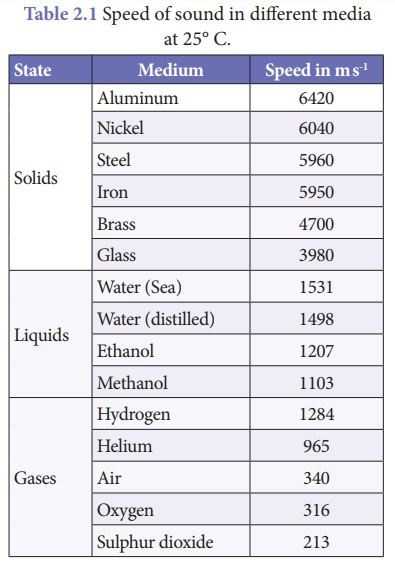Home | | Science 9th std | Speed of sound

# Speed of sound

The speed of sound is defined as the distance travelled by a sound wave per unit time as it propagates through an elastic medium.

Speed of sound

The speed of sound is defined as the distance travelled by a sound wave per unit time as it propagates through an elastic medium.If the distance traveled by one wave is taken as one wavelength (λ), and the time taken for this propagation is one time period (T), thenAs, T = 1/ n the speed (v) of sound is also written as, v = n λ

The speed of sound remains almost the same for all frequencies in a given medium under the same physical conditions.

### Example 1

A sound wave has a frequency of 2 kHz and wavelength of 15 cm. How much time will it take to travel 1.5 km?

### Solution:

Given, Frequency, n = 2 kHz = 2000Hz

Wavelength, λ = 15 cm = 0.15 m

Speed, v = n λ

= 0.15 × 2000 = 300 m s–1

The time taken (t) by the wave to travel a distance (d) of 1.5 km is calculated as, ­d = 1.5 km = 1500 m

Time (t) = Distance (d) / Velocity (v)

t = 1500/300 = 5 s

The sound will take 5 s to travel a distance of 1.5 km.

### Example 2

What is the wavelength of a sound wave in air at 20° C with a frequency of 22 MHz?

### Solution:

The speed of sound at 20° C is v = 344 m s-1.

The frequency of sound n = 22 MHz

= 22 × 106 Hz

To find the wavelength λ, we use the wave equation with speed of sound.

= v/n

= 344/22 × 106

= 15.64 × 10−6 m

Ans. λ = 15.64 µm.

## Speed of sound in differentmedia

Sound propagates through a medium at a finite speed. The sound of thunder is heard a little later than the flash of light is seen. So we can make out that sound travels with a speed which is much less than the speed of light. The speed of sound depends on the properties of the medium through which it travels.

The speed of sound in a gaseous medium depends on,

·           pressure of the medium

·           temperature of the medium

·           density of the medium

·           nature of gas

The speed of sound in solid medium depends on,

·           elastic property of the medium

·           temperature of the medium

·           density of the medium

The speed of sound is less in gaseous medium compared to solid medium. In any medium the speed of sound increases if we increase the temperature of the medium. For example the speed of sound in air is 330 m s–1 at 0°C and 340 m s–1 at 25°C. The speed of sound at a particular temperature in various media is listed in Table 2.1.Study Material, Lecturing Notes, Assignment, Reference, Wiki description explanation, brief detail
9th Science : Sound : Speed of sound |